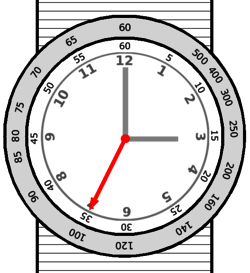On some of the analog watches or wristwatches from branch such as Omega and Casio, there is a outer scale bezel that inscribed around the rim and named tachymeter (also known as tachymetre or tacheometer). If you flip through the watch’s user guide or manual, you will not likely to find any explanation or definition on what is tachymeter or how to use the tachymeter functionality. Tachymeter is actually a tool used to compute speed based on travel time over a fixed distance traveled (like one mile or one kilometer). Thus, the tachymeter bezel or dial is a logarithmic scale (actually it’s not, as spacings between the marks on the tachymeter dial are proportional to 1/t where t is the elapsed time) that simplifies the computation of speed of an object, by computing the following function:

`Tachymeter Dial = 3600 / Elapsed Time In Seconds`Tachymeter illustration

Depending on on the range of the scale printed on the bezel, tachometer timer normally works and valid for all elapsed times from about 7.2 seconds to 60 seconds, thus only able to measure speed greater than 60 miles per hour or 60 kilometers per hour.

To use tachymeter, simply start the chronograph or stopwatch when the object measured passing the starting line. When the object reaches the next mile or kilometer marker, stop the chronograph or stopwatch. The point on the tachymeter scale that adjacent to the second hand of the watch indicating the speed (in miles per hour or kilometers per hour) of object traveling between the two points.

For example, we want to measure the average speed of a vehicle is moving. Start the chronometer or stopwatch function when the car passes the starting line, and stop the timer after the vehicle traveled exactly 1 mile or 1 kilometer. Then look at where the chronometer hand (the second hand of the watch) is pointing to, and get the reading or value of the corresponding number at the tachymeter bezel or dial. Let’s say the stopwatch stop at 3 o’clock position, meaning 15 seconds had elapsed for the car to travel 1 mile or 1 kilometer. At 3 o’clock position, the tachymetre value shows that number of 240, which means the average speed of the vehicle was 240 mph or km/h.

Due to the limitation and constraint on the tachymeter scale mentioned above which makes tachymetre measurements works and valid only for certain range of speed, so in order to calculate and measure slower speeds or higher speed, user can decrease or increase the unit of measurement (e.g. change to half-miles or half-kilometers, or ten miles or ten kilometres). However, simple calculation is needed in order to get the correct average speed by using this technique. It’s also true if the length of the distance does not exceed a unit of mile or kilometer, or other unit which the speed will be based on.

For example, if we want to measure how fast the 200m athlete is running. Start the chronometer when the race starts, and stop the stopwatch when athlete crosses the finishing line. Let’s say the athlete used 20 seconds to finish 200m running, so according to tachymeter, the speed of the athlete is 180 km/h. However, the athlete only ran one fifth (1/5) of a kilometer, so we should divide 180 with 5 or 1/5 of 180, which mean the actual speed of the runner is 36 km per hour.

The above example can be used for object or thing that moving too slowly too, as when the object travels 1 mile or 1 kilometer, the duration would have exceed 60 seconds, the maximum limit of tachymeter. So the possible workaround is to measure the time taken for the object to move a shorter distance (such as 100m) and divide the tachymeter value with 10 (as 100m is 1/10 of a kilometer).

What if the object travels too fast, such as a rocket or plane. In this case, user can increase the units of the distance covered by the object to measure a longer period of time so that the duration is greater than at least 7.2 seconds (typically the lower limit). We can measure the time taken by the object to cover 10 miles, let’s say it took 30 seconds, so the tachymeter will tell us the the speed is 120 miles per hour. However, 10 miles have been traveled, so that actual speed is 120 times 10, which is 1200 mph.

Wikipedia also explains that function performed by a tachymeter is independent of unit of distance (i.e. is not specific to miles or kilometers). A tachymeter can be used to measure speed in nautical miles per hour or meters per hour, or to measure an industrial production process in units per hour. A tachymeter is simply a means of converting elapsed time in seconds per X to X per hour.